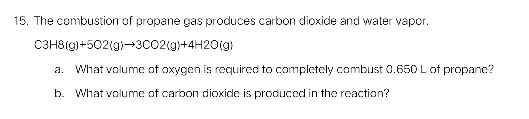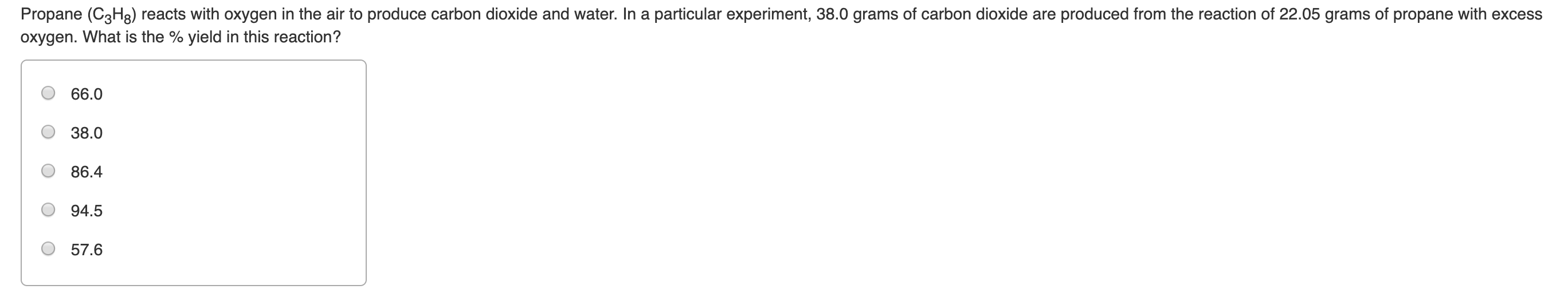Question

Consider the reaction below. If you start with 7.0 moles of C3H8 (propane) and 7.0 moles of O2, _ is the percent yield if 4.0 moles of carbon dioxide is produced.
C3H8(g) + 5O2(g) --> 3CO2(g) + 4H2O(g)

Answer 1

95%

#### Explanation:

The first thing that you need to do here is to figure out the theoretical yield of the reaction.

${\text{C"_ 3"H"_ (8(g)) + 5"O"_ (2(g)) -> 3"CO"_ (2(g)) + 4"H"_ 2"O}}_{\left(g\right)}$

You know that the reaction consumes $5$ moles of oxygen gas for every $1$ mole of propane that takes part in the reaction.

This means that, in order for the reaction to consume $7$ moles of propane, it must also consume

7.0 color(red)(cancel(color(black)("moles C"_3"H"_8))) * "5 moles O"_2/(1color(red)(cancel(color(black)("moles C"_3"H"_8)))) = "35 moles O"_2

Since you have only $7$ moles of oxygen gas, you can say that oxygen gas will be the limiting reagent, i.e. it will be completely consumed before all the moles of propane will get the chance to react.

So the reaction will consume $7$ moles of oxygen gas and

7.0 color(red)(cancel(color(black)("moles O"_2))) * ("1 mole C"_3"H"_8)/(5color(red)(cancel(color(black)("moles O"_2)))) = "1.4 moles C"_3"H"_8

In this case, you can say that the reaction can theoretically produce, i.e. what you would get at 100% yield

7 color(red)(cancel(color(black)("moles O"_2))) * "3 moles CO"_2/(5color(red)(cancel(color(black)("moles O"_2)))) = "4.2 moles CO"_2

However, you know that the actual yield of the reaction is $4.1$ moles of carbon dioxide, so you can say that the percent yield of the reaction was

"% yield" = (4.0 color(red)(cancel(color(black)("moles CO"_2))))/(4.2color(red)(cancel(color(black)("moles CO"_2)))) * 100% = color(darkgreen)(ul(color(black)(95%)))

The answer is rounded to two sig figs.

#### Earn Coins

Coins can be redeemed for fabulous gifts.

Similar Homework Help Questions
• ### How molecules of CO2 (g) are produced when 0.75 moles of propane are burned? Chemical reaction:...

How molecules of CO2 (g) are produced when 0.75 moles of propane are burned? Chemical reaction: C3H8 + 5O2 à 3CO2 + 4H2O

• ### Many home barbeques are fueled with propane gas C3H8

Many home barbeques are fueled with propane gas C3H8.What mass of carbon dioxide (in kg) is produced upon the complete combustion of 18.9L  of propane (approximate contents of one 5-gallon tank)? Assume that the density of the liquid propane in the tank is 0.621g/ml.How do I start & work this question?I wrote a balanced equation:C3H8 + 5O2 -> 4H2O + 3CO2Now, where do I go from here?

• ### Calculate the amount of carbon dioxide produced when burning 10.0 g of propane with 5 g...

Calculate the amount of carbon dioxide produced when burning 10.0 g of propane with 5 g of oxygen in the following reaction.             C3H8      +      5 O2     ------->      3 CO2       +      4 H2O

• ### I am still not quite grasping how to complete this problem: Posted by K on Sunday, October 14, 2007 at 6:43pm

I am still not quite grasping how to complete this problem:Posted by K on Sunday, October 14, 2007 at 6:43pm. Many home barbeques are fueled with propane gas C3H8. What mass of carbon dioxide (in kg) is produced upon the complete combustion of 18.9L of propane (approximate contents of one 5-gallon tank)? Assume that the density of the liquid propane in the tank is 0.621g/ml. How do I start & work this question? I wrote a balanced equation: C3H8 +...

• ### What is the percent yield of carbon dioxide if the reaction of 74.4 g of carbon...

What is the percent yield of carbon dioxide if the reaction of 74.4 g of carbon monoxide produces 88.4 g of carbon dioxide? Iron(III)(III) oxide reacts with carbon monoxide to produce iron and carbon dioxide. Fe2O3(s)+3CO(g)→2Fe(s)+3CO2(g)

• ### 2. A normal dose of ibuprofen is 200. mg. How many ibuprofen molecules are there in...

2. A normal dose of ibuprofen is 200. mg. How many ibuprofen molecules are there in a dose of ibuprofen? The molecular formula of ibuprofen is C13H18O2. (Hint: grams to mol and mol to molecules) 3-Propane fuel is used in BBQs. Use the following reaction to determine how many moles of carbon dioxide gas are produced from burning of 6 moles of propane gas. C3H8(g) + 5O2(g) ®  4H2O(g) + 3CO2(g) 4- A. If a person inhales 51 moles of oxygen,...

• ### ( a) In the complete combustion of propane, how many moles of H2O(l) are produced per mole of CO2(g)? (b) A 1.638-g sample of propane is burned in excess oxygen. What are the theoretical yields (in grams) of CO2(g) and H2O(l) expected from the reaction

C3H8(g)+5O2(g)→ 3CO2(g)+4H2O(l)

• ### 15. The combustion of propane gas produces carbon dioxide and water vapor C3H8ig+502(g)3CO2(g+4H20(g) What volume of...15. The combustion of propane gas produces carbon dioxide and water vapor C3H8ig+502(g)3CO2(g+4H20(g) What volume of oxygen is required to completely combust 0.650 L of propane? a. b. What volume of carbon dioxicle is produced in the reaction?

• ### Propane (C3Hg) reacts with oxygen in the air to produce carbon dioxide and water. In a...Propane (C3Hg) reacts with oxygen in the air to produce carbon dioxide and water. In a particular experiment, 38.0 grams of carbon dioxide are produced from the reaction of 22.05 grams of propane with excess oxygen. What is the % yield in this reaction? 66.0 38.0 86.4 94.5 57.6